http www comp nus edu sgcs 1010 UNIT

• Slides: 48http: //www. comp. nus. edu. sg/~cs 1010/ UNIT 3 Overview of C Programming© NUS CS 1010 (AY 2014/5 Semester 1) Unit 3 - 2 Unit 3: Overview of C Programming Objectives: § Learn basic C constructs, interactive input, output, § § and arithmetic operations Learn some data types and the use of variables to hold data Understand basic programming style References: § Chapter 2 Variables, Arithmetic Expressions and § Input/Output Chapter 3 Lessons 3. 1 Math Library Functions and 3. 2 Single Character Data© NUS CS 1010 (AY 2014/5 Semester 1) Unit 3: Overview of C Programming 1. A Simple C Program 2. Variables and Data Types 3. Program Structure § § Preprocessor directives Input Compute Output 4. Math Functions 5. Programming Style 6. Common Mistakes Unit 3 - 3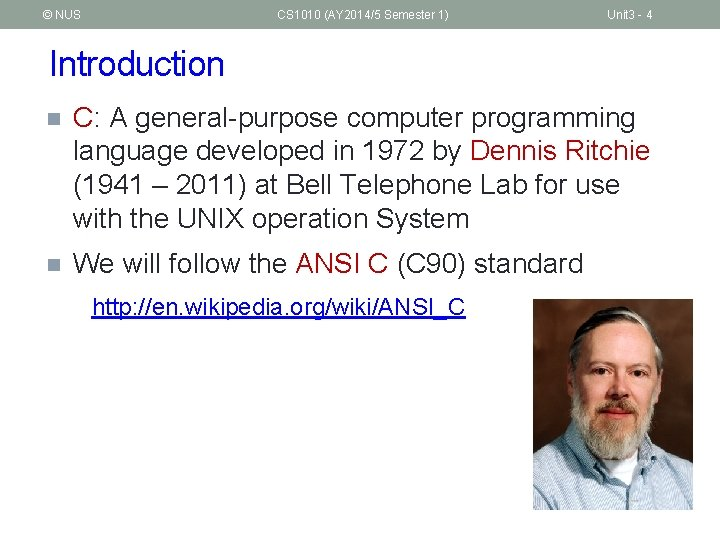© NUS CS 1010 (AY 2014/5 Semester 1) Unit 3 - 4 Introduction n C: A general-purpose computer programming language developed in 1972 by Dennis Ritchie (1941 – 2011) at Bell Telephone Lab for use with the UNIX operation System n We will follow the ANSI C (C 90) standard http: //en. wikipedia. org/wiki/ANSI_C© NUS CS 1010 (AY 2014/5 Semester 1) Unit 3 - 5 Quick Review: Edit, Compile, Execute Edit Compile Test, test, and test! Edit produces eg: vim first. c Compile eg: a. out first. c Executable code produces eg: gcc first. c Execute Source code produces a. out Program output The value of c is 3. Cannot compile? Incorrect result?© NUS CS 1010 (AY 2014/5 Semester 1) Unit 3 - 6 A Simple C Program (1/3) n General form of a simple C program preprocessor directives main function header { declaration of variables executable statements } “Executable statements” usually consists of 3 parts: § § § Input data Computation Output results© NUS CS 1010 (AY 2014/5 Semester 1) A Simple C Program (2/3) Unit 3 - 7 Unit 3_Mile. To. Km. c // Converts distance in miles to kilometres. #include <stdio. h> /* printf, scanf definitions */ #define KMS_PER_MILE 1. 609 /* conversion constant */ int main(void) { float miles, kms; // input – distance in miles // output – distance in kilometres /* Get the distance in miles */ printf("Enter distance in miles: "); scanf("%f", &miles); // Convert the distance to kilometres kms = KMS_PER_MILE * miles; // Display the distance in kilometres printf("That equals %9. 2 f km. n", kms); return 0; } Sample run \$ gcc –Wall Week 2_Mile. To. Km. c \$ a. out Enter distance in miles: 10. 5 That equals 16. 89 km.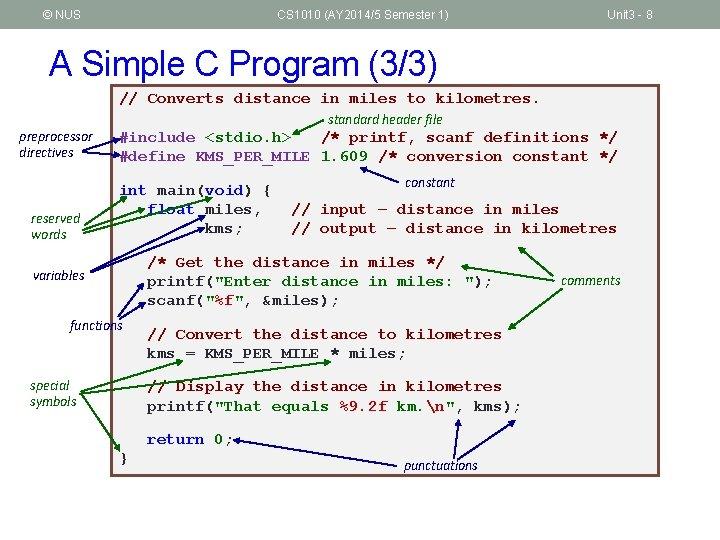© NUS CS 1010 (AY 2014/5 Semester 1) Unit 3 - 8 A Simple C Program (3/3) // Converts distance in miles to kilometres. preprocessor directives reserved words standard header file #include <stdio. h> /* printf, scanf definitions */ #define KMS_PER_MILE 1. 609 /* conversion constant */ int main(void) { float miles, kms; constant // input – distance in miles // output – distance in kilometres /* Get the distance in miles */ printf("Enter distance in miles: "); scanf("%f", &miles); variables functions special symbols // Convert the distance to kilometres kms = KMS_PER_MILE * miles; // Display the distance in kilometres printf("That equals %9. 2 f km. n", kms); return 0; } punctuations comments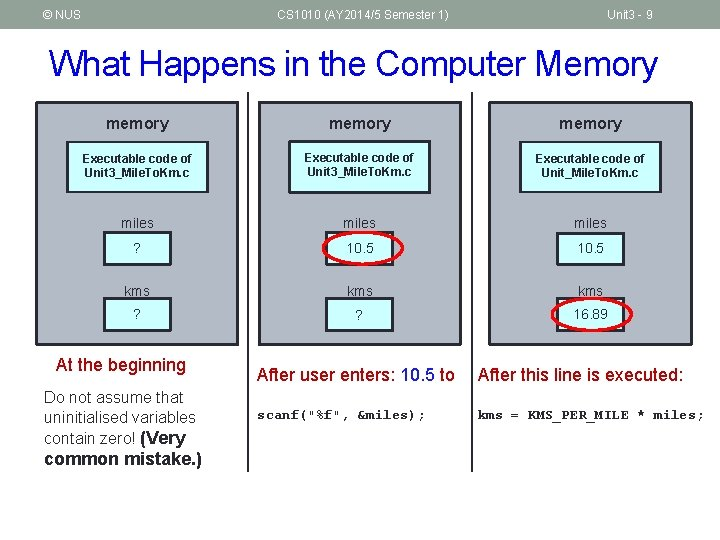© NUS CS 1010 (AY 2014/5 Semester 1) Unit 3 - 9 What Happens in the Computer Memory memory Executable code of Unit 3_Mile. To. Km. c Executable code of Unit_Mile. To. Km. c miles ? 10. 5 kms kms ? ? 16. 89 At the beginning Do not assume that uninitialised variables contain zero! (Very common mistake. ) After user enters: 10. 5 to After this line is executed: scanf("%f", &miles); kms = KMS_PER_MILE * miles;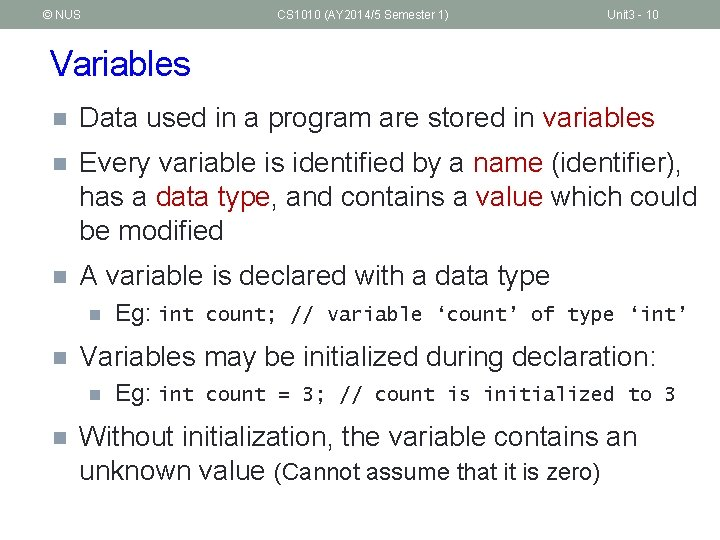© NUS CS 1010 (AY 2014/5 Semester 1) Unit 3 - 10 Variables n Data used in a program are stored in variables n Every variable is identified by a name (identifier), has a data type, and contains a value which could be modified n A variable is declared with a data type n n Variables may be initialized during declaration: n n Eg: int count; // variable ‘count’ of type ‘int’ Eg: int count = 3; // count is initialized to 3 Without initialization, the variable contains an unknown value (Cannot assume that it is zero)© NUS CS 1010 (AY 2014/5 Semester 1) Unit 3 - 11 Variables: Mistakes in Initialization n Incorrect: No initialization int count; count = count + 12; n Does ‘count’ contain 12 after this statement? Redundant initialization int count = 0; count = 123; Initialization here is redundant.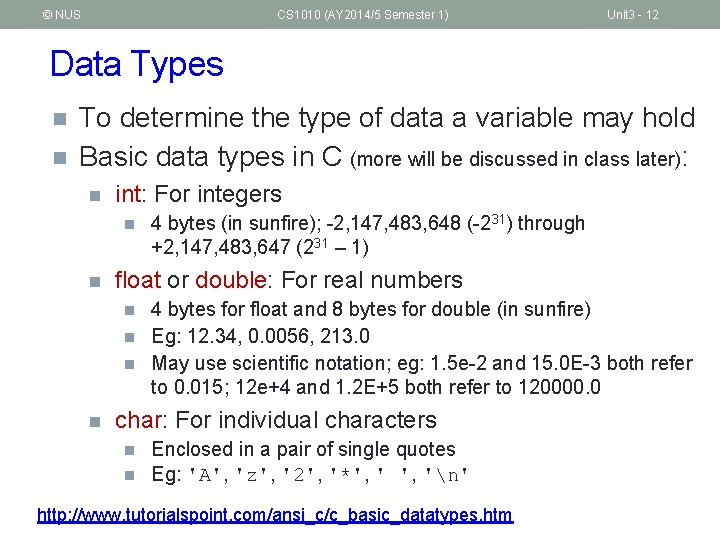© NUS CS 1010 (AY 2014/5 Semester 1) Unit 3 - 12 Data Types n n To determine the type of data a variable may hold Basic data types in C (more will be discussed in class later): n int: For integers n n float or double: For real numbers n n 4 bytes (in sunfire); -2, 147, 483, 648 (-231) through +2, 147, 483, 647 (231 – 1) 4 bytes for float and 8 bytes for double (in sunfire) Eg: 12. 34, 0. 0056, 213. 0 May use scientific notation; eg: 1. 5 e-2 and 15. 0 E-3 both refer to 0. 015; 12 e+4 and 1. 2 E+5 both refer to 120000. 0 char: For individual characters n n Enclosed in a pair of single quotes Eg: 'A', 'z', '2', '*', 'n' http: //www. tutorialspoint. com/ansi_c/c_basic_datatypes. htm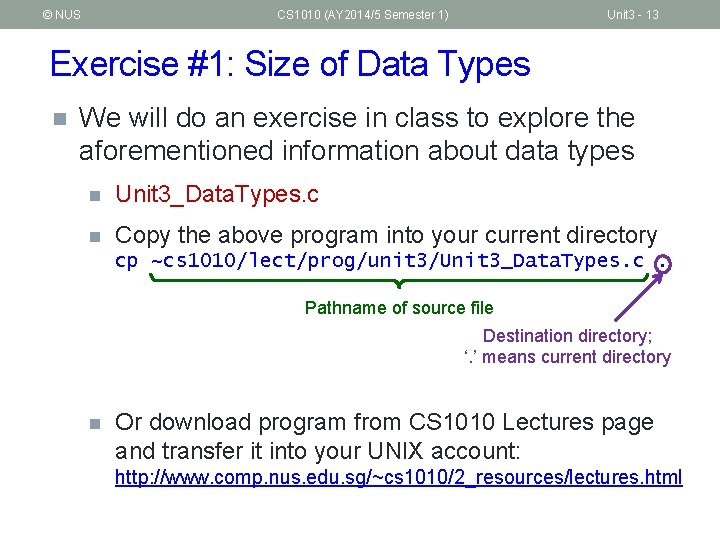© NUS CS 1010 (AY 2014/5 Semester 1) Unit 3 - 13 Exercise #1: Size of Data Types n We will do an exercise in class to explore the aforementioned information about data types n Unit 3_Data. Types. c n Copy the above program into your current directory cp ~cs 1010/lect/prog/unit 3/Unit 3_Data. Types. c. Pathname of source file Destination directory; ‘. ’ means current directory n Or download program from CS 1010 Lectures page and transfer it into your UNIX account: http: //www. comp. nus. edu. sg/~cs 1010/2_resources/lectures. html© NUS CS 1010 (AY 2014/5 Semester 1) Unit 3 - 14 Notes (1/2) n Basic steps of a simple program 1. Read inputs (scanf) 2. Compute 3. Print outputs (printf) n For now we will use interactive inputs n n Standard input stream (stdin) – default is keyboard Use the scanf() function Assume input data always follow specification n Hence no need to validate input data (for now) Outputs n n Standand output stream (stdout) – default is monitor Use the printf() function© NUS CS 1010 (AY 2014/5 Semester 1) Unit 3 - 15 Notes (2/2) n Include header file <stdio. h> to use scanf() and printf() n n Read n n Include the header file (for portability sake) even though some systems do no require this to be done Lessons 1. 6 – 1. 9 Important! (Code. Crunch issue) n Make sure you have a newline character (‘n’) at the end of your last line of output, or Code. Crunch may mark your output as incorrect. printf("That equals %9. 2 f km. n", kms);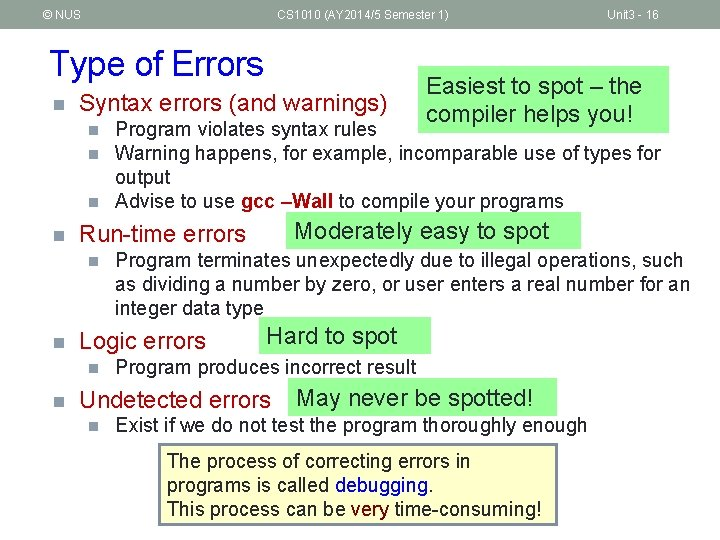© NUS CS 1010 (AY 2014/5 Semester 1) Type of Errors n Syntax errors (and warnings) n n n Moderately easy to spot Program terminates unexpectedly due to illegal operations, such as dividing a number by zero, or user enters a real number for an integer data type Logic errors n Hard to spot Program produces incorrect result Undetected errors n Easiest to spot – the compiler helps you! Program violates syntax rules Warning happens, for example, incomparable use of types for output Advise to use gcc –Wall to compile your programs Run-time errors n Unit 3 - 16 May never be spotted! Exist if we do not test the program thoroughly enough The process of correcting errors in programs is called debugging. This process can be very time-consuming!© NUS CS 1010 (AY 2014/5 Semester 1) Unit 3 - 17 Program Structure n A basic C program has 4 main parts: n Preprocessor directives: n n eg: #include <stdio. h>, #include <math. h>, #define PI 3. 142 Input: through stdin (using scanf), or file input We will learn Compute: through arithmetic operations file input/output Output: through stdout (using printf), or file output later.© NUS CS 1010 (AY 2014/5 Semester 1) Unit 3 - 18 Program Structure: Preprocessor Directives (1/2) n The C preprocessor provides the following Input Compute Output n Inclusion of header files n Macro expansions Conditional compilation For now, we will focus on inclusion of header files and simple application of macro expansions n n n Preprocessor Inclusion of header files n To use input/output functions such as scanf() and printf(), you need to include <stdio. h>: #include <stdio. h> n To use mathematical functions, you need to include <math. h>: #include <math. h>© NUS CS 1010 (AY 2014/5 Semester 1) Unit 3 - 19 Program Structure: Preprocessor Directives (2/2) n Preprocessor Macro expansions n n Input Compute Output One of the uses is to define a macro for a constant value Eg: #define PI 3. 142 // use all CAP for macro #define PI 3. 142 Preprocessor replaces all instances of PI with 3. 142 before passing the program to the compiler. int main(void) {. . . area. Circle = PI * radius; vol. Cone = PI * radius * height / 3. 0; } What the compiler sees: int main(void) {. . . area. Circle = 3. 142 * radius; vol. Cone = 3. 142 * radius * height / 3. 0; }© NUS CS 1010 (AY 2014/5 Semester 1) Unit 3 - 20 Program Structure: Input/Output (1/3) § Input/output statements: § printf ( format string, print list ); § printf ( format string ); § scanf( format string, input list ); One version: age 20 Preprocessor Input Compute Output Address of variable ‘age’ varies each time a program is run. “age” refers to value in the variable age. “&age” refers to (address of) the memory point cell where the value of age is stored. int age; double cap; // cumulative average printf("What is your age? "); scanf("%d", &age); printf("What is your CAP? "); scanf("%lf", &cap); printf("You are %d years old, and your CAP is %fn", age, cap); Another version: Unit 3_Input. Output. c int age; Unit 3_Input. Output. V 2. c double cap; // cumulative average point printf("What are your age and CAP? "); scanf("%d %lf", &age, &cap); printf("You are %d years old, and your CAP is %fn", age, cap);© NUS CS 1010 (AY 2014/5 Semester 1) Unit 3 - 21 Program Structure: Input/Output (2/3) Preprocessor Input Compute Output § %d and %lf are examples of format specifiers; they are placeholders for values to be displayed or read Placeholder Variable Type Function Use %c char printf / scanf %d int printf / scanf %f float or double printf %f float scanf %lf double scanf %e float or double printf (for scientific notation) § Examples of format specifiers used in printf(): § § %5 d: to display an integer in a width of 5, right justified %8. 3 f: to display a real number (float or double) in a width of 8, with 3 decimal places, right justified § See Table 2. 3 (page 65) for sample displays § Note: For scanf(), just use the format specifier without indicating width, decimal places, etc.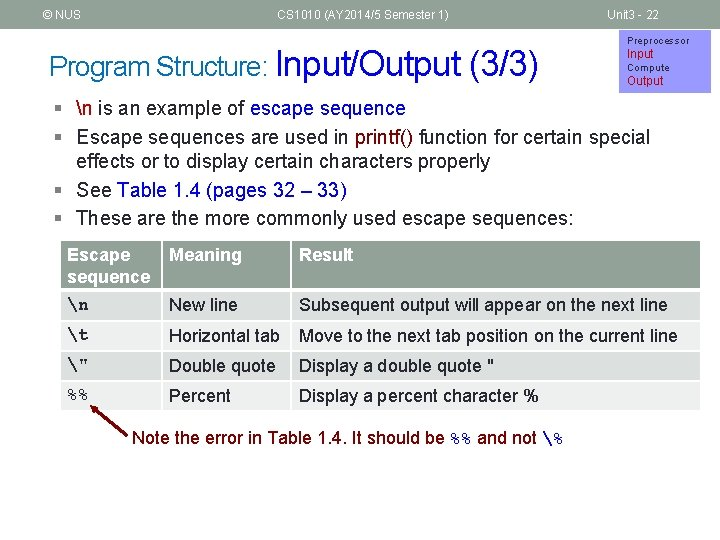© NUS CS 1010 (AY 2014/5 Semester 1) Program Structure: Input/Output (3/3) Unit 3 - 22 Preprocessor Input Compute Output § n is an example of escape sequence § Escape sequences are used in printf() function for certain special effects or to display certain characters properly § See Table 1. 4 (pages 32 – 33) § These are the more commonly used escape sequences: Escape sequence Meaning Result n New line Subsequent output will appear on the next line t Horizontal tab Move to the next tab position on the current line " Double quote Display a double quote " %% Percent Display a percent character % Note the error in Table 1. 4. It should be %% and not %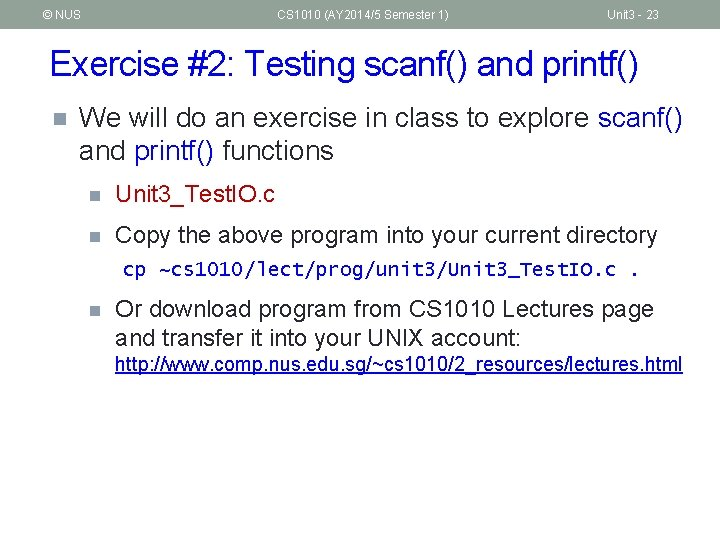© NUS CS 1010 (AY 2014/5 Semester 1) Unit 3 - 23 Exercise #2: Testing scanf() and printf() n We will do an exercise in class to explore scanf() and printf() functions n Unit 3_Test. IO. c n Copy the above program into your current directory cp n ~cs 1010/lect/prog/unit 3/Unit 3_Test. IO. c. Or download program from CS 1010 Lectures page and transfer it into your UNIX account: http: //www. comp. nus. edu. sg/~cs 1010/2_resources/lectures. html© NUS CS 1010 (AY 2014/5 Semester 1) Unit 3 - 24 Exercise #3: Distance Conversion (1/2) n Convert distance from miles to kilometres n Unit 3_Mile. To. Km. c n The program is given (which you can copy to your directory as earlier instructed), but for this exercise we want you to type in the program yourself as a practice in using vim n The program is shown in the next slide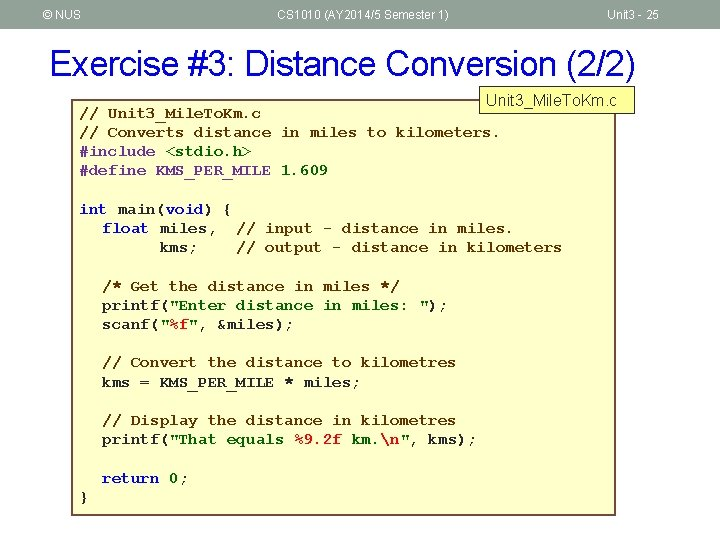© NUS CS 1010 (AY 2014/5 Semester 1) Unit 3 - 25 Exercise #3: Distance Conversion (2/2) Unit 3_Mile. To. Km. c // Converts distance in miles to kilometers. #include <stdio. h> #define KMS_PER_MILE 1. 609 int main(void) { float miles, // input - distance in miles. kms; // output - distance in kilometers /* Get the distance in miles */ printf("Enter distance in miles: "); scanf("%f", &miles); // Convert the distance to kilometres kms = KMS_PER_MILE * miles; // Display the distance in kilometres printf("That equals %9. 2 f km. n", kms); return 0; }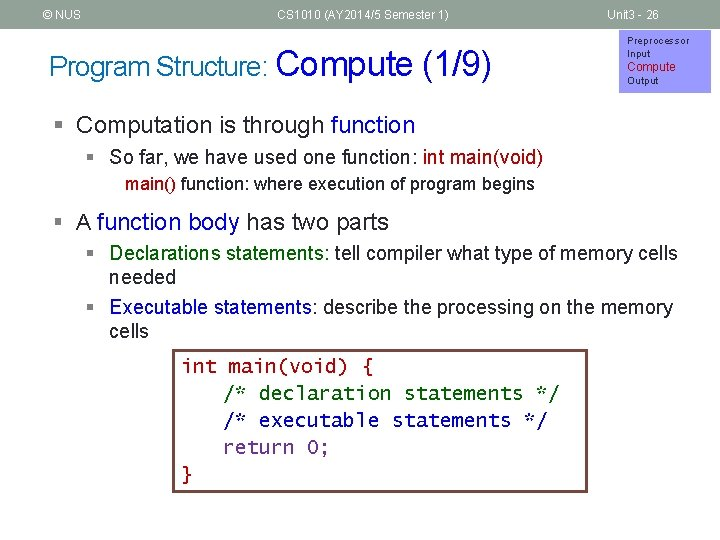© NUS CS 1010 (AY 2014/5 Semester 1) Program Structure: Compute (1/9) Unit 3 - 26 Preprocessor Input Compute Output § Computation is through function § So far, we have used one function: int main(void) main() function: where execution of program begins § A function body has two parts § Declarations statements: tell compiler what type of memory cells needed § Executable statements: describe the processing on the memory cells int main(void) { /* declaration statements */ /* executable statements */ return 0; }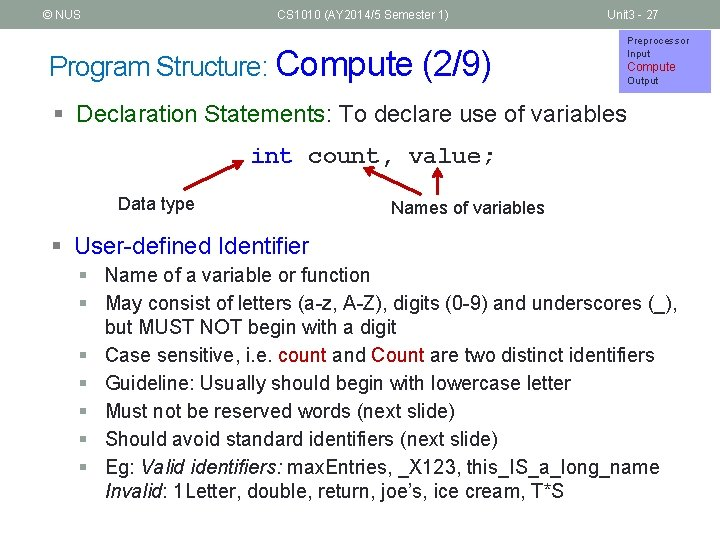© NUS CS 1010 (AY 2014/5 Semester 1) Program Structure: Compute (2/9) Unit 3 - 27 Preprocessor Input Compute Output § Declaration Statements: To declare use of variables int count, value; Data type Names of variables § User-defined Identifier § Name of a variable or function § May consist of letters (a-z, A-Z), digits (0 -9) and underscores (_), but MUST NOT begin with a digit § Case sensitive, i. e. count and Count are two distinct identifiers § Guideline: Usually should begin with lowercase letter § Must not be reserved words (next slide) § Should avoid standard identifiers (next slide) § Eg: Valid identifiers: max. Entries, _X 123, this_IS_a_long_name Invalid: 1 Letter, double, return, joe’s, ice cream, T*S© NUS CS 1010 (AY 2014/5 Semester 1) Program Structure: Compute (3/9) Unit 3 - 28 Preprocessor Input Compute Output § Reserved words (or keywords) § § Have special meaning in C Eg: int, void, double, return Complete list: http: //c. ihypress. ca/reserved. html Cannot be used for user-defined identifiers (names of variables or functions) § Standard identifiers § Names of common functions, such as printf, scanf § Avoid naming your variables/functions with the same name of built -in functions you intend to use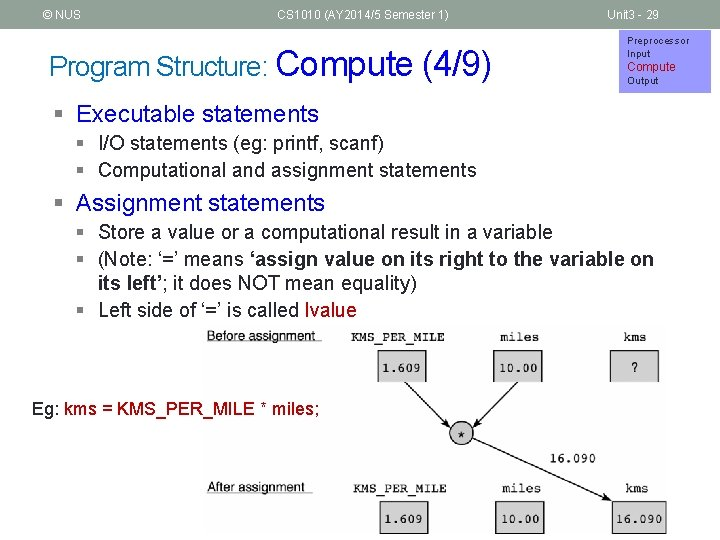© NUS CS 1010 (AY 2014/5 Semester 1) Program Structure: Compute (4/9) Unit 3 - 29 Preprocessor Input Compute Output § Executable statements § I/O statements (eg: printf, scanf) § Computational and assignment statements § Assignment statements § Store a value or a computational result in a variable § (Note: ‘=’ means ‘assign value on its right to the variable on its left’; it does NOT mean equality) § Left side of ‘=’ is called lvalue Eg: kms = KMS_PER_MILE * miles;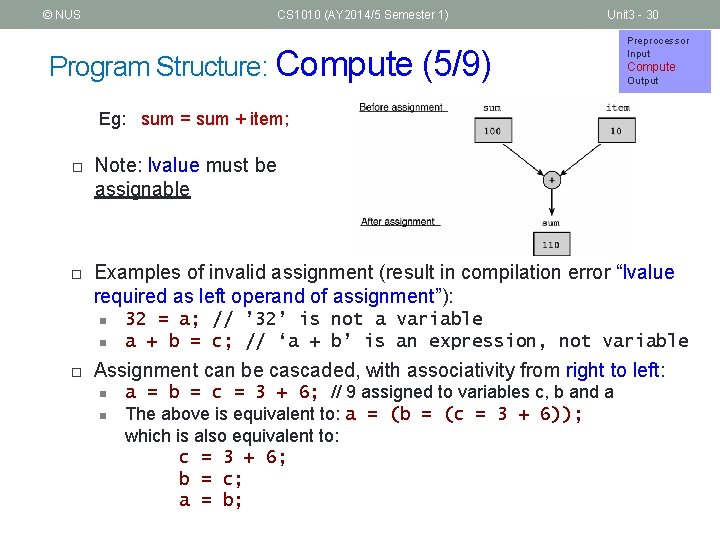© NUS CS 1010 (AY 2014/5 Semester 1) Unit 3 - 30 Program Structure: Compute (5/9) Preprocessor Input Compute Output Eg: sum = sum + item; ¨ ¨ Note: lvalue must be assignable Examples of invalid assignment (result in compilation error “lvalue required as left operand of assignment”): n n ¨ 32 = a; // ’ 32’ is not a variable a + b = c; // ‘a + b’ is an expression, not variable Assignment can be cascaded, with associativity from right to left: n n a = b = c = 3 + 6; // 9 assigned to variables c, b and a The above is equivalent to: a = (b = (c = 3 + 6)); which is also equivalent to: c = 3 + 6; b = c; a = b;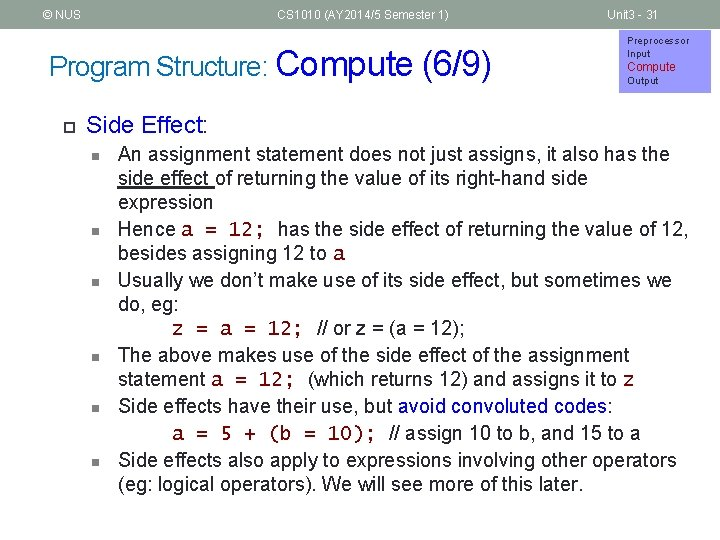© NUS CS 1010 (AY 2014/5 Semester 1) Program Structure: Compute (6/9) ¨ Unit 3 - 31 Preprocessor Input Compute Output Side Effect: n n n An assignment statement does not just assigns, it also has the side effect of returning the value of its right-hand side expression Hence a = 12; has the side effect of returning the value of 12, besides assigning 12 to a Usually we don’t make use of its side effect, but sometimes we do, eg: z = a = 12; // or z = (a = 12); The above makes use of the side effect of the assignment statement a = 12; (which returns 12) and assigns it to z Side effects have their use, but avoid convoluted codes: a = 5 + (b = 10); // assign 10 to b, and 15 to a Side effects also apply to expressions involving other operators (eg: logical operators). We will see more of this later.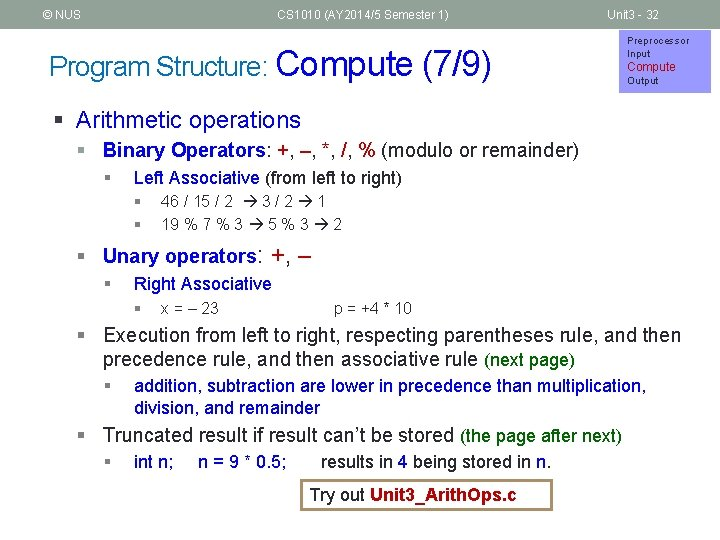© NUS CS 1010 (AY 2014/5 Semester 1) Unit 3 - 32 Program Structure: Compute (7/9) Preprocessor Input Compute Output § Arithmetic operations § Binary Operators: +, –, *, /, % (modulo or remainder) § Left Associative (from left to right) § § 46 / 15 / 2 3 / 2 1 19 % 7 % 3 5 % 3 2 § Unary operators: +, – § Right Associative § x = – 23 p = +4 * 10 § Execution from left to right, respecting parentheses rule, and then precedence rule, and then associative rule (next page) § addition, subtraction are lower in precedence than multiplication, division, and remainder § Truncated result if result can’t be stored (the page after next) § int n; n = 9 * 0. 5; results in 4 being stored in n. Try out Unit 3_Arith. Ops. c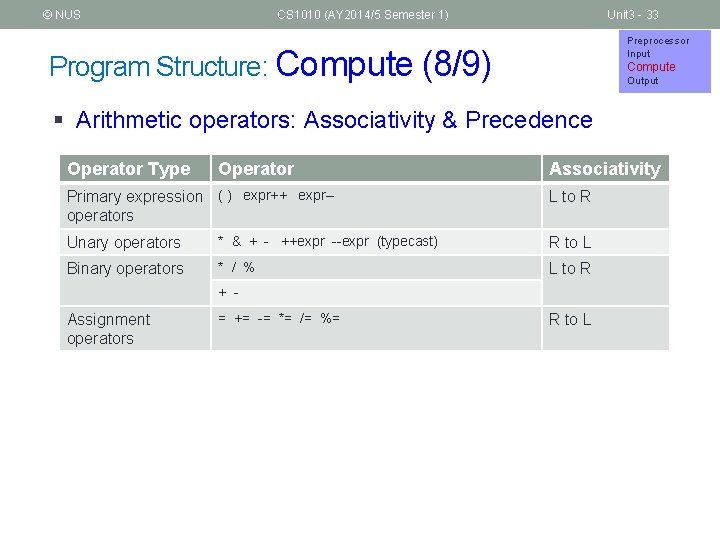© NUS CS 1010 (AY 2014/5 Semester 1) Unit 3 - 33 Preprocessor Input Program Structure: Compute (8/9) Compute Output § Arithmetic operators: Associativity & Precedence Operator Type Operator Primary expression ( ) expr++ expr-operators Associativity L to R Unary operators * & + - ++expr --expr (typecast) R to L Binary operators * / % L to R + - Assignment operators = += -= *= /= %= R to L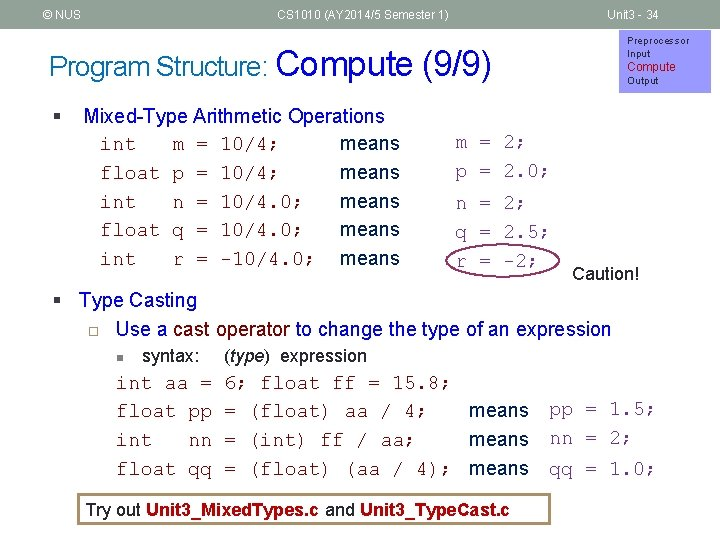© NUS CS 1010 (AY 2014/5 Semester 1) Unit 3 - 34 Preprocessor Input Program Structure: Compute (9/9) § Mixed-Type Arithmetic Operations int m = 10/4; means float p = 10/4; means int n = 10/4. 0; means float q = 10/4. 0; means int r = -10/4. 0; means Compute Output m = 2; p = 2. 0; n = 2; q = 2. 5; r = -2; Caution! § Type Casting ¨ Use a cast operator to change the type of an expression n syntax: (type) expression int aa = float pp int nn float qq 6; float ff = 15. 8; = (float) aa / 4; means pp = 1. 5; = (int) ff / aa; means nn = 2; = (float) (aa / 4); means qq = 1. 0; Try out Unit 3_Mixed. Types. c and Unit 3_Type. Cast. c© NUS CS 1010 (AY 2014/5 Semester 1) Unit 3 - 35 Exercise #4: Temperature Conversion n Instructions will be given out in class n We will use this formula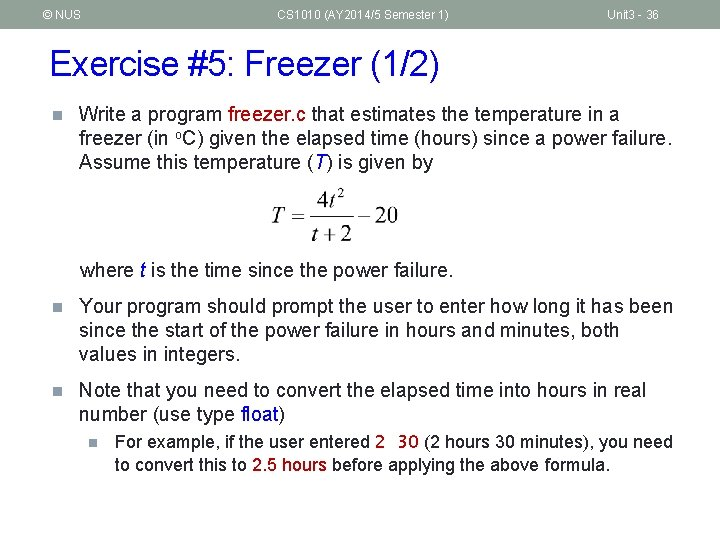© NUS CS 1010 (AY 2014/5 Semester 1) Unit 3 - 36 Exercise #5: Freezer (1/2) n Write a program freezer. c that estimates the temperature in a freezer (in o. C) given the elapsed time (hours) since a power failure. Assume this temperature (T) is given by where t is the time since the power failure. n Your program should prompt the user to enter how long it has been since the start of the power failure in hours and minutes, both values in integers. n Note that you need to convert the elapsed time into hours in real number (use type float) n For example, if the user entered 2 30 (2 hours 30 minutes), you need to convert this to 2. 5 hours before applying the above formula.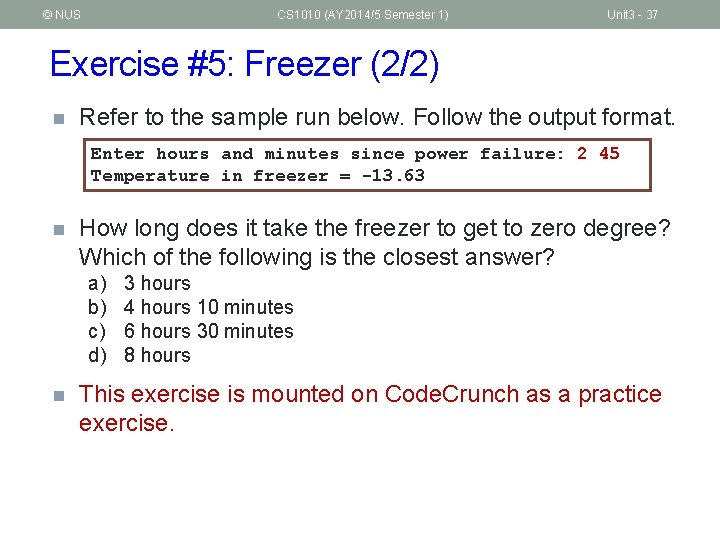© NUS CS 1010 (AY 2014/5 Semester 1) Unit 3 - 37 Exercise #5: Freezer (2/2) n Refer to the sample run below. Follow the output format. Enter hours and minutes since power failure: 2 45 Temperature in freezer = -13. 63 n How long does it take the freezer to get to zero degree? Which of the following is the closest answer? a) b) c) d) n 3 hours 4 hours 10 minutes 6 hours 30 minutes 8 hours This exercise is mounted on Code. Crunch as a practice exercise.© NUS CS 1010 (AY 2014/5 Semester 1) Unit 3 - 38 Math Functions (1/2) n In C, there are many libraries offering functions for you to use. n Eg: scanf() and printf() – requires to include <stdio. h> n In Exercise #5, for t 2 you may use t*t, or the pow() function in the math library: pow(t, 2) n n To use math functions, you need to n n n pow(x, y) // computes x raised to the power of y Include <math. h> AND Compile your program with –lm option (i. e. gcc –lm …) See Tables 3. 3 and 3. 4 (pages 88 – 89) for some math functions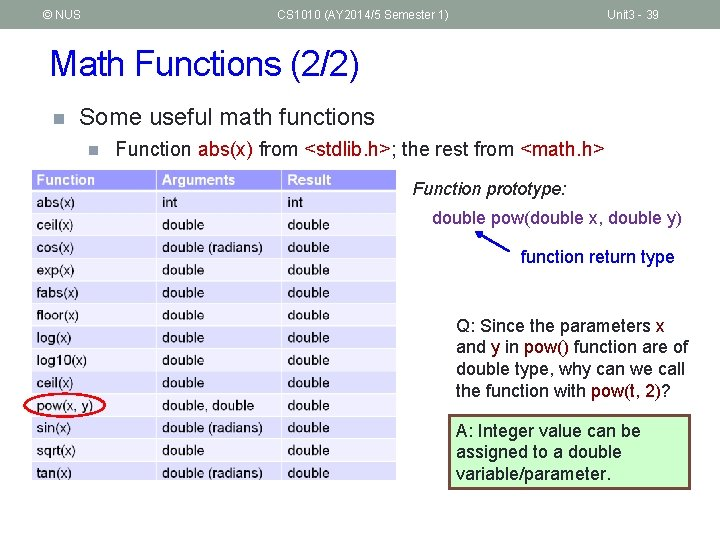© NUS CS 1010 (AY 2014/5 Semester 1) Unit 3 - 39 Math Functions (2/2) n Some useful math functions n Function abs(x) from <stdlib. h>; the rest from <math. h> Function prototype: double pow(double x, double y) function return type Q: Since the parameters x and y in pow() function are of double type, why can we call the function with pow(t, 2)? A: Integer value can be assigned to a double variable/parameter.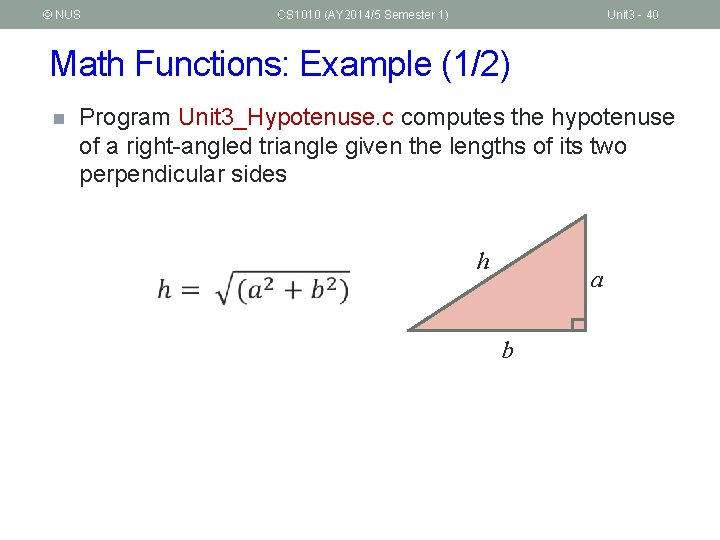© NUS CS 1010 (AY 2014/5 Semester 1) Unit 3 - 40 Math Functions: Example (1/2) n Program Unit 3_Hypotenuse. c computes the hypotenuse of a right-angled triangle given the lengths of its two perpendicular sides h a b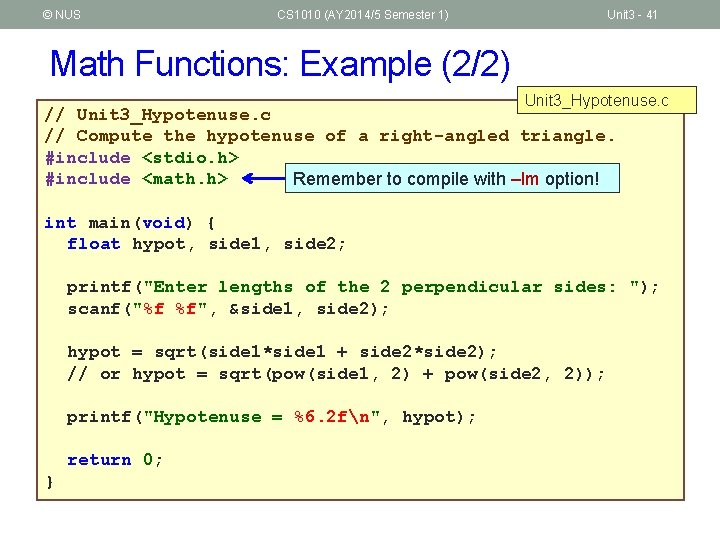© NUS CS 1010 (AY 2014/5 Semester 1) Unit 3 - 41 Math Functions: Example (2/2) Unit 3_Hypotenuse. c // Compute the hypotenuse of a right-angled triangle. #include <stdio. h> #include <math. h> Remember to compile with –lm option! int main(void) { float hypot, side 1, side 2; printf("Enter lengths of the 2 perpendicular sides: "); scanf("%f %f", &side 1, side 2); hypot = sqrt(side 1*side 1 + side 2*side 2); // or hypot = sqrt(pow(side 1, 2) + pow(side 2, 2)); printf("Hypotenuse = %6. 2 fn", hypot); return 0; }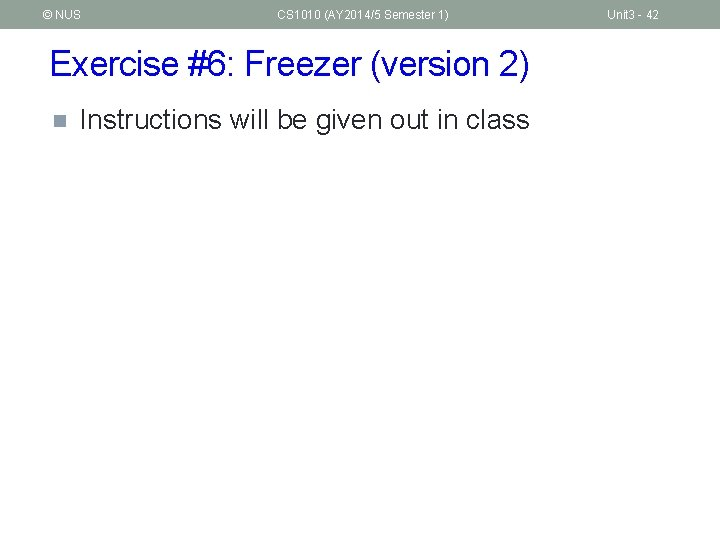© NUS CS 1010 (AY 2014/5 Semester 1) Exercise #6: Freezer (version 2) n Instructions will be given out in class Unit 3 - 42© NUS CS 1010 (AY 2014/5 Semester 1) Unit 3 - 43 Programming Style (1/2) n Programming style is just as important as writing a correct program n Refer to some C Style Guides on the CS 1010 website http: //www. comp. nus. edu. sg/~cs 1010/2_resources/online. html n In your lab assignments, marks will be awarded to style besides program correctness n n n Correctness: 60% Style: 20% Design: 20%© NUS CS 1010 (AY 2014/5 Semester 1) Unit 3 - 44 Programming Style (2/2) n Identifier naming for variables and functions n n n User-defined constants n n n Use lower-case with underscore or capitalise first character of every subsequent word (Eg: celsius, sum, second_max, second. Max; NOT Celsius, SUM, Second. Max) Must be descriptive (Eg: num. Years instead of ny, abc, xbrt) Use upper-case with underscore (Eg: KMS_PER_MILE, DAYS_IN_YEAR) Consistent indentation Appropriate comments Spacing and blank lines And many others In vim, typing gg=G would auto-indent your program nicely!© NUS CS 1010 (AY 2014/5 Semester 1) Unit 3 - 45 Common Mistakes (1/2) § Not initialising variables EXTREMELY COMMON MISTAKE § Program may work on some machine but not on another! Cannot assume that the initial value of b is zero! int a, b; a = b + 3; // but what is the value of b? § Unnecessary initialisation of variables int x = 0; x = 531; int x = 0; scanf("%d", &x); § Forgetting & in a scanf() statement int x; scanf("%d", x); int x; scanf("%d", &x);© NUS CS 1010 (AY 2014/5 Semester 1) Unit 3 - 46 Common Mistakes (2/2) § Forgetting to compile with –lm option when the program uses math functions. § Forgetting to recompile after modifying the source code. Sometimes when your program crashes, a “core dump” may happen. Remove the file “core” (UNIX command: rm core) from your directory as it takes up a lot of space.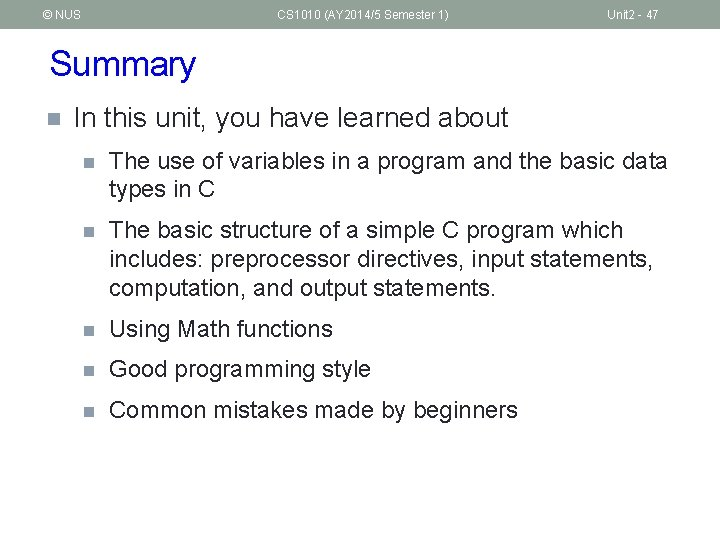© NUS CS 1010 (AY 2014/5 Semester 1) Unit 2 - 47 Summary n In this unit, you have learned about n The use of variables in a program and the basic data types in C n The basic structure of a simple C program which includes: preprocessor directives, input statements, computation, and output statements. n Using Math functions n Good programming style n Common mistakes made by beginners© NUS CS 1010 (AY 2014/5 Semester 1) End of File Unit 3 - 48